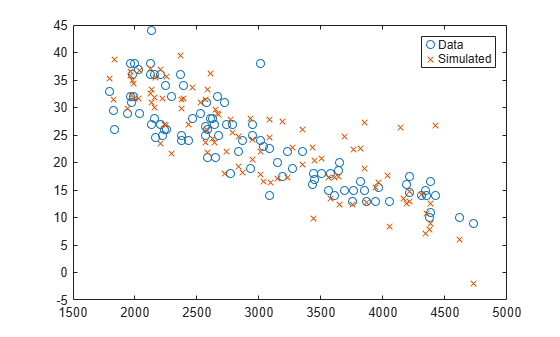# random

Class: NonLinearModel

Simulate responses for nonlinear regression model

## Syntax

```ysim = random(mdl) ysim = random(mdl,Xnew) ysim = random(mdl,Xnew,'Weights',W) ```

## Description

`ysim = random(mdl)` simulates responses from the fitted nonlinear model `mdl` at the original design points.

`ysim = random(mdl,Xnew)` simulates responses from the fitted nonlinear model `mdl` to the data in `Xnew`, adding random noise.

`ysim = random(mdl,Xnew,'Weights',W)` simulates responses using the observation weights, `W`.

## Input Arguments

 `mdl` Nonlinear regression model, constructed by `fitnlm`. `Xnew` Points at which `mdl` predicts responses. If `Xnew` is a table or dataset array, it must contain the predictor names in `mdl`.If `Xnew` is a numeric matrix, it must have the same number of variables (columns) as was used to create `mdl`. Furthermore, all variables used in creating `mdl` must be numeric. `W` Vector of real, positive value weights or a function handle. If you specify a vector, then it must have the same number of elements as the number of observations (or rows) in `Xnew`.If you specify a function handle, the function must accept a vector of predicted response values as input, and returns a vector of real positive weights as output. Given weights, `W`, `random` estimates the error variance at observation `i` by `MSE*(1/W(i))`, where MSE is the mean squared error. Default: No weights

## Output Arguments

 `ysim` Vector of predicted mean values at `Xnew`, perturbed by random noise. The noise is independent, normally distributed, with mean zero, and variance equal to the estimated error variance of the model.

## Examples

expand all

Create a nonlinear model of car mileage as a function of weight, and simulate the response.

Create an exponential model of car mileage as a function of weight from the `carsmall` data. Scale the weight by a factor of 1000 so all the variables are roughly equal in size.

```load carsmall X = Weight; y = MPG; modelfun = 'y ~ b1 + b2*exp(-b3*x/1000)'; beta0 = [1 1 1]; mdl = fitnlm(X,y,modelfun,beta0);```

Create simulated responses to the data.

```Xnew = X; ysim = random(mdl,Xnew);```

Plot the original responses and the simulated responses to see how they differ.

```plot(X,y,'o',X,ysim,'x') legend('Data','Simulated')```## Alternatives

For predictions without added noise, use `predict`.